#### 期刊菜单

Research on Trajectory Tracking Control for Coal Gangue Sorting Robot Arm
DOI: 10.12677/AIRR.2022.114045, PDF, HTML, XML, 下载: 98  浏览: 157

Abstract: During the picking process of the coal gangue sorting robotic arm, external factors such as buffeting of coal gangue on the belt conveyor impact the end of the manipulator, which leads to the uncertainty of the control system of the manipulator, and causes the control system when the manipulator grabs the coal gangue. The performance is degraded and the trajectory tracking error is too large; for this reason, based on the traditional sliding mode control, an RBF neural network switching gain adjustment sliding mode control method is improved. According to the Lagrangian theory, the dynamic mathematical model of the coal gangue sorting manipulator is deduced. For the uncertain part of the model, the sliding mode control algorithm and the RBF neural network are combined, and the RBF neural network is used to adjust the switching gain in the sliding mode control. The switching gain can be changed with the change of the uncertain part, while reducing the chattering problem in the traditional synovial control. Using MATLAB software, the controller simulation experiment was carried out, and the tracking effect of the joint angle of the manipulator and the response time of the switching gain adjustment were analyzed. The simulation results show that the control method described in this paper can approach the desired trajectory value faster, and the tracking error is also reduced. Build an experimental platform, carry out experiments on the coal gangue sorting robotic arm, and simulate the zigzag path of sorting coal gangue. According to the feedback data of the laser calibrator, it is concluded that the control method can ensure the rationality and safety of the movement of the manipulator, and can achieve the desired trajectory in the coal gangue sorting process by convergence in a short time, which is in line with condition needs of the actual work of coal gangue sorting.

1. 引言

2. 机械臂动力学建模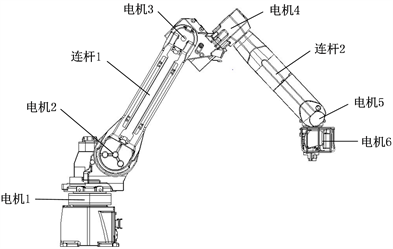Figure 1. Coal gangue sorting robotic arm structure

$M\left(q\right)\stackrel{¨}{q}+C\left(q,\stackrel{˙}{q}\right)\stackrel{˙}{q}+F\left(\stackrel{˙}{q}\right)+G\left(q\right)=\tau -{\tau }_{d}$ (1)

${m}_{1}{‖x‖}^{2}\le {x}^{\text{T}}D\left(q\right)x\le {m}_{2}{‖x‖}^{2}$ (2)

$M\left(q\right)\stackrel{¨}{q}+C\left(q,\stackrel{˙}{q}\right)+G\left(q\right)=u\left(t\right)$ (3)

$\left\{\begin{array}{l}M\left(q\right)={M}_{0}\left(q\right)+\Delta M\left(q\right)\\ C\left(q,\stackrel{˙}{q}\right)={C}_{0}\left(q,\stackrel{˙}{q}\right)+\Delta C\left(q,\stackrel{˙}{q}\right)\\ G\left(q\right)={G}_{0}\left(q\right)+\Delta G\left(q\right)\end{array}$ (4)

$\Delta h=\Delta M\left(q\right)\stackrel{¨}{q}+\Delta C\left(q,\stackrel{˙}{q}\right)\stackrel{˙}{q}+\Delta G\left(q\right)$ (5)

${M}_{0}\left(q\right)\stackrel{¨}{q}+{C}_{0}\left(q,\stackrel{˙}{q}\right)\stackrel{˙}{q}+{G}_{0}\left(q\right)={\tau }_{eq}$ (6)

${M}_{0}\left(q\right)\stackrel{¨}{q}+{C}_{0}\left(q,\stackrel{˙}{q}\right)\stackrel{˙}{q}+{G}_{0}\left(q\right)+\Delta h+{\tau }_{d}={\tau }_{eq}+{\tau }_{s}=\tau$ (7)

3. 基于RBF网络增益调节的滑膜控制

3.1. 增益滑膜控制器的设计

$e=q-{q}_{d}$ (8)

$s\left(x,t\right)=ce+\stackrel{˙}{e}$ (9)

${\tau }_{eq}={M}_{0}\left(q\right)\left({\stackrel{¨}{q}}_{d}+\stackrel{˙}{e}+e\right)+{C}_{0}\left(q,\stackrel{˙}{q}\right)\stackrel{˙}{q}+{G}_{0}\left(q\right)$ (10)

$\stackrel{¨}{e}={M}_{0}^{-1}\left(q\right)\left(\Delta h+{\tau }_{d}-{\tau }_{s}\right)-\stackrel{˙}{e}-e$ (11)

$s=\stackrel{˙}{e}+Ce$ (12)

$\stackrel{˙}{s}=\stackrel{¨}{e}+C\stackrel{˙}{e}={M}_{0}^{-1}\left(q\right)\left(\Delta h+{\tau }_{d}\right)-{M}_{0}^{-1}\left(q\right){\tau }_{s}-\stackrel{˙}{e}-e$ (13)

$\stackrel{˙}{s}=-\xi \mathrm{sgn}\left(s\right)-ks$ (14)

${\tau }_{s}={M}_{0}\left(q\right)\left(\xi \mathrm{sgn}\left(s\right)+ks+C\stackrel{˙}{e}-\stackrel{˙}{e}-e\right)$ (15)

3.2. 采用RBF网络增益调节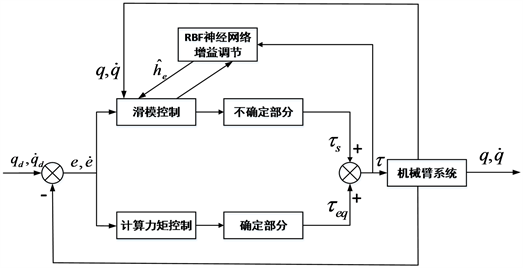Figure 2. Neural network gain adjustment control system

${\stackrel{^}{h}}_{e}={\stackrel{^}{W}}^{\text{T}}\phi \left(x\right)$ (16)

$\Delta h-{\stackrel{^}{h}}_{e}={W}^{\text{T}}\phi \left(x\right)-{\stackrel{^}{W}}^{\text{T}}\phi \left(x\right)+\xi$ (17)

$K=|{w}^{\text{T}}h\left(x\right)|$ (18)

${h}_{i}\left(x\right)=\mathrm{exp}\left(-\frac{{‖x-{m}_{i}‖}^{2}}{{\sigma }_{i}^{2}}\right)$

$E=\frac{1}{2}{e}^{2}$ (19)

$\stackrel{^}{W}=Fh{s}^{\text{T}}{M}_{0}^{-1}$ (20)

${M}_{0}\left(q\right)\stackrel{¨}{q}+{C}_{0}\left(q,\stackrel{˙}{q}\right)\stackrel{˙}{q}+{G}_{0}\left(q\right)+{\stackrel{^}{W}}^{\text{T}}\phi =\tau$ (21)

$\stackrel{˙}{s}=-\xi \mathrm{sgn}\left(s\right)-ks+{M}_{0}^{-1}\left({\stackrel{^}{W}}^{\text{T}}\phi \left(x\right)+\xi \right)$ (22)

$\tau ={\tau }_{eq}+{\tau }_{s}={M}_{0}\left(q\right)\left({\stackrel{¨}{q}}_{d}+\xi \mathrm{sgn}\left(s\right)+ks+C\stackrel{˙}{e}\right)+{C}_{0}\left(q,\stackrel{˙}{q}\right)+{G}_{0}\left(q\right)+{\stackrel{^}{W}}^{\text{T}}\phi \left(x\right)$ (23)

$V=\frac{1}{2}{s}^{\text{T}}s+\frac{1}{2}tr\left({\stackrel{˜}{W}}^{\text{T}}{F}^{-1}\stackrel{˜}{W}\right)$ (24)

$\stackrel{˙}{V}={s}^{\text{T}}\left(-\xi \mathrm{sgn}\left(s\right)-ks\right)+{s}^{\text{T}}\left({M}_{0}^{-1}\xi \right)\le {s}^{\text{T}}\left(-\xi \mathrm{sgn}\left(s\right)-ks\right)\le 0$ (25)

4. 仿真分析

4.1. 工况场景描述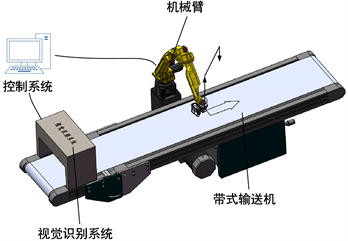Figure 3. Schematic diagram of coal gangue mechanical arm sorting system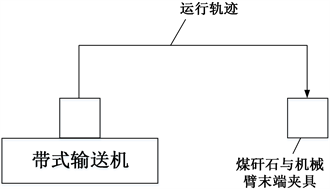Figure 4. Schematic diagram of sorting track

4.2. MATLAB仿真分析

${w}_{i}={\left[\begin{array}{cccccc}20& 20& 20& 20& 20& 20\end{array}\right]}^{\text{T}}$，其中 $i=1~6$

${\delta }_{1}\left(t\right){\left[\begin{array}{cccccc}20& 20& 20& 20& 20& 20\end{array}\right]}^{\text{T}}$，其中 $i=1~6$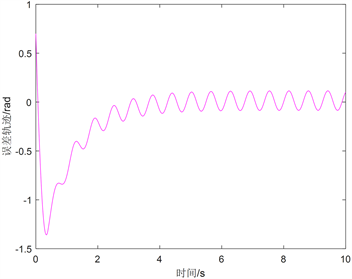(a) 传统滑模控制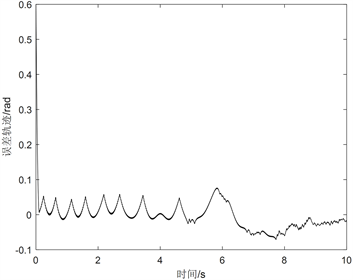(b) 基于切换增益调节的神经网络滑模控制

Figure 5. Tracking error response curve of sinusoidal trajectory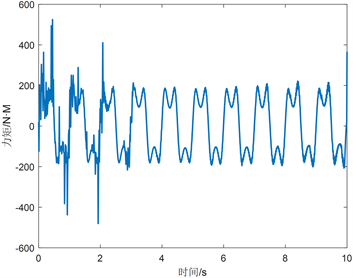(a) 传统滑模控制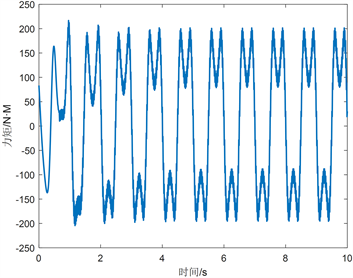(b) 基于切换增益调节的神经网络滑模控制

Figure 6. Sinusoidal variation control input response curve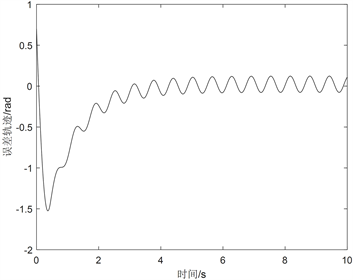(a) 传统滑模控制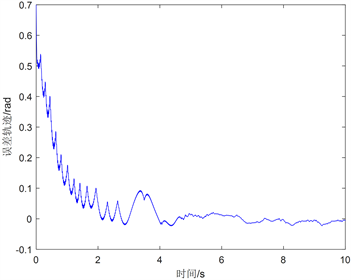(b) 基于切换增益调节的神经网络滑模控制

Figure 7. Tracking error response curve of ramp change trajectory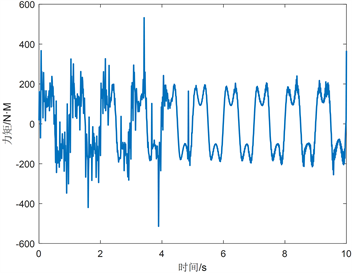(a) 传统滑模控制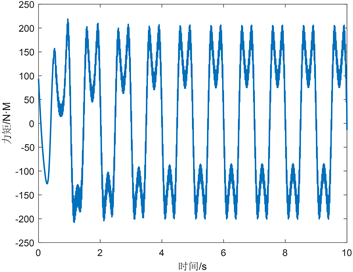(b) 基于切换增益调节的神经网络滑模控制

Figure 8. Ramp change control input response curve(a) 传统滑模控制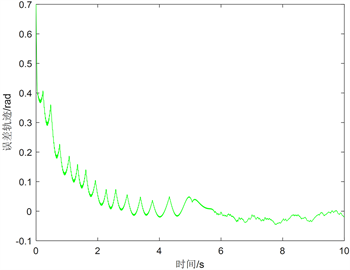(b) 基于切换增益调节的神经网络滑模控制

Figure 9. Step change trajectory tracking error response curve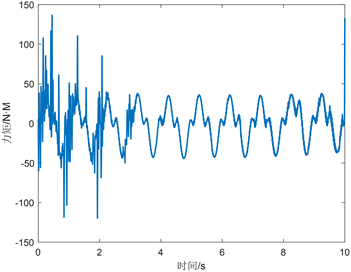(a) 传统滑模控制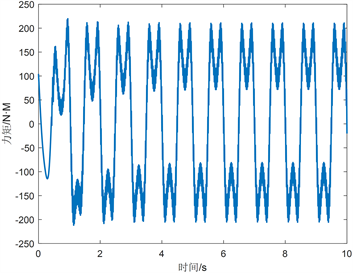(b) 基于切换增益调节的神经网络滑模控制

Figure 10. Step change control input response curve

5. 实验分析

5.1. 实验平台搭建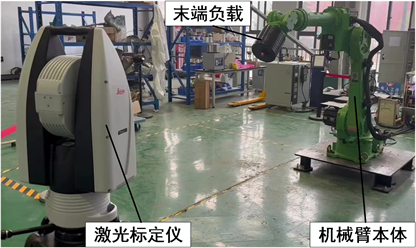Figure 11. Coal gangue sorting robotic arm experimental platform

5.2. 结果分析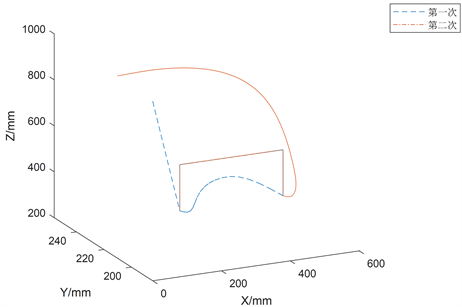Figure 12. Robot arm end curve

6. 结论

1) 研究煤矸石分拣机械臂分拣煤矸石过程中轨迹跟踪控制，针对煤矸石分拣机械臂关节位置，提出一种基于RBF神经网络切换增益调节滑模控制器；实现对控制系统中不确定性部分的实时补偿调节，达到提高控制系统精度、控制响应速度的目的。